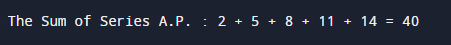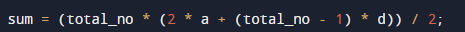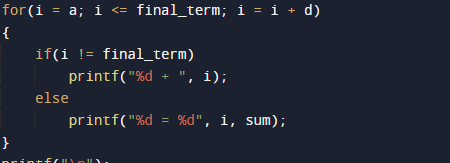C Program To find sum of AP series

In this tutorial you will learn about the C Program To find sum of AP series and its application with practical example.

In this tutorial, we will learn to create a C program that will find sum of AP Series  using C programming.

Prerequisites

Before starting with this tutorial we assume that you are best aware of the following C programming topics:

• Basic C programming.
• C loop statements
• C for Loop.
• Basic input/output.
• Conditional statement.
• If-else statement.

What Is Arithmetic Progression series?

A Series with same constant difference is known as arithmetic series. This constant is called  common difference.The first element of series is  a  and constant difference is d. The series is looks like a, a + d, a + 2d, a + 3d,a+4d . . no.

Example.

If a=1 and d=3 and total_term=5C Program To find sum of AP series

In this program we will print A.P series using for loop. We would first declared and initialized the required variables. Next, we would prompt user to input the number of terms. Later we will generate the A.P series of specified number of terms and sum of terms.

Final_term of  series:

In the above program, we have first declared and initialized a set variables required in the program.

• Total_no= number of terms to be printed.
• d = common difference.
• final_term= Last term of series.
• i=  for iteration.
• sum = adding sum of series.

In the next statement user will be prompt to enter the first term,common difference and total term in the series in following variable total_no,a,d .
The formula used in this program is.Where Total_no is the last term of a finite sequence and d is common difference and sum the sum of Total_no terms.
Then, we will find the sum of the A.P Series. Here, we used  Loop to display the A.P series.You can see in image how series  printing and showing the sum or series.

In this tutorial we have learn about the C Program To find sum of AP series and its application with practical example. I hope you will like this tutorial.